# Short Notes: Solutions# Types of Solutions

Depending on the types of solvents and its combination with the solutes, there may be various types of solutions.

When solvent is Gas

1. Gas + Gas e.g.- Mixture of Gases, CO2 in air, N2 in air.
2. Gas + Liquid e.g.- Moisture (water vapour), mist
3. Gas + solid. e.g.- Sublimation of solid in air like I2 , NH4Cl Camphor, Carbon in air (smoke)

When Solvent is Liquid

1. Liquid + Gas e.g.- Dissolved O2 in water or Soda Water (dissolved CO2 in Water).
2. Liquid + Liquid e.g.- Alcohol or Acetone in water
3. Liquid + solid e.g.- salt or sugar in water.

When solvent is Solid

1. Solid + Gas e.g. – Solution of hydrogen in Nickel.
2. Solid + liquid e.g.- Sodium amalgam with Mercury
3. Solid + Solid e.g.- Alloys (Brass, German Silver, Bronze)

# Methods of Expressing the concentration of Solutions in different units

There are various methods by which we can describe the concentration of a solution quantitatively ?

(i) Mass percentage (w/w): ? Here solute and solvent both are expressed in weight or mass.

Mass % of a component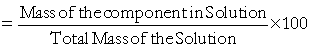For example, 10% glucose in water means, 10g glucose dissolved in 90g water resulting 100g of solutions.

(ii)Volume Percentage (v/v):? Here solute and solvent both are expressed in volume.

Volume % of a Component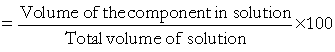For example, 10% alcohol solution in water means 10ml alcohol is dissolved in 90 ml of water resulting 100 ml of total solutions.

(iii) Mass by volume percentage (w/v): ? Here solute is expressed in its weight while the solvent is expressed in its volume.

Mass ?Volume % =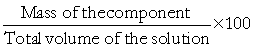For example, If we say that 10% of salt (w/v) is dissolved in water, it only means that 10 g of salt is dissolved in 100 ml of water, but not in 90 ml of water. This unit is commonly used in medicine and pharmacy.

(iv) Parts per million (ppm):- When the relative quantity of solute in a solution is very low (i.e. in trace), it is difficult to express it in the normal scale. Here we estimate number of solute particles per million ( 106 ).

Parts per million (ppm)ppm can also be expressed in m/m, v/v and m/v as in the case of concentration in percentage. This unit is commonly used environmental chemistry and pharmacy.

? Concentration in percentage can be converted to ppm by multiplying it by 104 .

? For example, if we say that 0.08% of oil is present in a contaminated water sample it means 0.08 × 104 = 800 ppm oil is in the water sample.

(v) Mole fraction (x) :- Mole fraction of a component (solute or solvent) in a solution is expressed as .

Mole fraction of a Component (x) =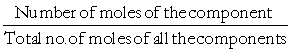Unit of mole fraction (x) being a ratio is dimensionless.

For example, in a binary solution (containing two components A & B). if

nA = number of moles of A

nB = number of moles of B.

Mole fraction of A and B can be expressed as ?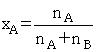Similarly,For a solution containing i no. of components. We have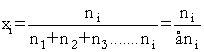It is easy to show that in a given solution sum of mole fractions of all components is equal to unity (i.e. 1).

x1 + x2 + x3 ………+ xi = 1

Example 1: What will be the mole fraction of ethylene glycol ( C2H6O2 ) in a solution containing 20% of ( C2H6O2 ) by mass?

Solution: Here we assume that we have 100 g of solution. Solution will contain 20 g of ethylene glycol and 80 g of water.(vi) Molarity (M):- It is the number of moles of a solute dissolved in one litre (or one dm3) of solution.Example 2: What will be the molarity of a solution containing 5 g of NaOH in 450 mL solution?

Solution:(vii) Molality (m): It is the number of moles of solute dissolved per kg. of solvent.(viii) Normality (N):– It is the number of gram equivalent weight of solute dissolved per litre of the solution.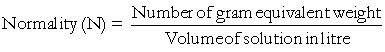We should recall, Number of gram equivalent weight of a substance is the molar mass divided by its acidity (in case of base) or basicity (in case of acid) or charges (ve or + ve) present on it in case of ionic compound.

# Solubility

The maximum amount of substance (solute) that can be dissolved in a specific amount of a particular solvent at a certain temperature is called the solubility of the substance in that solvent.

Solubility depends on the intermolecular interactions between the solute and solvent as well as temperature and pressure. Let us consider these factors how effect the solution of solid and gas in a liquid.

(i) Solubility of solid in a liquid: It is law of nature that like dissolves like. This means that polar solutes dissolve in polar solvents and non-polar solutes in non polar solvents. For example, sodium chloride and sugar dissolve readily in water but not in benzene. On the other hand naphthalene and authracene being non-polar solute can readily dissolve in non-polar solvent like benzene but not in polar solvent like water.

Dissolution:- When a solid solute starts dissolving in a solvent its concentration increases in solution, this process is called dissolution.

Crystallization:- Some solute particles in solution collide with undissolved solid solute and get separated out of solution, the process is called crystallization.

Saturation Point:- At dynamic equilibrium, (when dissolution and crystallization occur at the same rate) the concentration of solution is found to be constant and no more solute can be dissolved at a given temperature and pressure. This stage of solution is called saturation point. The saturation point varies in different types of solution.

Effect of Pressure: – Since the solids and liquids are not compressible, practically there is no effect of pressure on the solubility of a solid in a liquid.

Effect of Temperature:- Effect of temperature on solubility of a solid in a liquid at dynamic equilibrium must follow Le chateliers Principle, according to which, In a nearly saturated solution.

if dissolution process is endothermic (ΔsolH>O) , the solubility should increase with rise in temperature.

(a) If dissolution process is exothermic (ΔsolH<O) the solubility should decrease with rise in temperature.

Also, experimentally the two conditions are followed.

(ii)Solubility of a Gas in a liquid: Many gases dissolve in water. O2 Dissolves only to a small extent in water. Because of dissolved oxygen in water all aquatic life is possible. On the other hand Hydrogen chloride gas (HCl) is highly soluble in water because of its ion formation.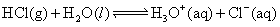Solubility of gases in liquids is greatly affected by pressure and temperature.

Effect of Pressure:- Effect of pressure on the solubility of gas in a liquid was first explained by Henry. He gave a quantitative relation between pressure and solubility.From above figure here we see that the concentration of dissolved gases increases with the increase in the pressure on the gas above the solution

Henry’s Law: At constant temperature the partial pressure of the gas in vapour phase (p) is proportional to the mole fraction of the gas (x) in the solution. It is expressed as

p = KHx

K= Henry’s constant

Different gases have different  KH values at the same temperature, hence K is a function of nature of gas.

From above equation it is clear that at a given pressure higher the value of K lower is the mole fraction and hence lower the solubility.

Effect of Temperature: Gas molecules, when dissolved in liquid, are in liquid phase and the dissolution process is like condensation which is exothermic (heat evolving). According to Le chatelier’s principle, solubility of gas into liquid being an exothermic process should decrease with increase in temperature.

## Vapour Pressure of Liquid solutions

(i) Vapour pressure of liquid-liquid solutions and Rault’s Law:

If Ptotal = total vapour pressure

P1 = partial vapour pressure of component 1.

P2 = partial vapour pressure of component 2.

The quantitative relationship between the partial pressures ( P1 , P2 ) and the corresponding mole fractions ( x1, x2 ) of the two components 1 & 2 respectively, was given by Marte Raoult known as Raoult’s law which states ?

Raoult’s law: ?For a solution of volatile liquids, the partial vapour pressure of each component in the solution is directly proportional to its mole fraction.?

Thus, for component 1,

p1 ∝ x1

or, p1 = p10x1

Similarly, for component 2,

p2 = p20x2

Where, p1and p20= vapour pressures of the pure component 1 and 2 respectively at same temperature.

#### From Dalton?s Law of Partial PressureSimilarly if we put the value (x2 = 1− x1) in equation ptotal = x1p10 + x2p20 it can be shown easily that

ptotal = p20 + (p10 − p20)x1

##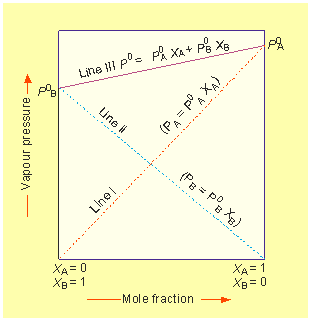#### The above plot shows that the plot of p1 or p2 verses the mole fractions x1 & x2  for a solution is linear hence p1 & p2 are directly proportional to x1 & x2 respectively.

In Figure, the dashed lines I & II represent the partial pressures of components, while the marked Line III represents the total vapour pressure of the solution.

Conclusions from Raoult’s law:

1. Total vapour pressure over the solution can be related to the mole fractions of any one component.

i.e. ptotal = p10 + (p20 − p10)x2 = p20 + (p10 − p20)x1

2. Total vapour pressure over the solution varies linearly with the mole fraction of component 2.

3. Depending on the vapour pressures of the pure components 1 and 2, total vapour pressure over the solution decreases or increases with the increase of the mole fraction of component 1. (See above plot)

Does Raoult’s law differ from Henry’s law to some extent?

Exactly not, only the two cases are different and we can say that Raoult’s law is a special case of Henry’s law in which kH becomes equal to p10.

Raoult’s law describes liquid-liquid solutions in which one component is volatile while Henry’s law describes the solution in which one component so volatile that it exists as a gas i.e. the solution of a gas in liquid.

p = p0x     Raoult’s law

p = kHx      Henry’s law

The equation of Raoult’s law & Henry’s law say same thing that the vapour pressure of a volatile component or a gas is directly proportional to its mole fraction in a solution. Only the proportionality constant in case of Raoult’s law is p0 while it is kH in case of Henry’s law, which may or may not be equal to p0 .

(ii) Vapour pressure of solutions of solids in Liquids: We know from unit 5 (class XI) that when liquids vapourise at a certain temperature, a pressure is exerted by the vapour of liquid over the liquid phase at equilibrium conditions, known as vapour pressure of that liquid.

In a pure liquid the entire surface is filled by only liquid molecules, but when we add a non-volatile solute to a liquid solution, a part of the liquid surface is also occupied by these solutes. This reduces the space to the liquid molecules to evaporate out resulting the lowering in vapour pressure because only solvent part of the solution can vapourise and creates vapour pressure, but not the solute part being non-volatile.

#### KEY CONCEPT

Decrease in vapour pressure due to presence of non-volatile solute in a solution doesn’t depend on the nature of solute but on the quantity of solute.

Experimentally it is observed that decrease in vapour pressure of water by adding 1 mol of sucrose to 1 kg water is nearly same to that by 1 mol of urea to 1 kg water.

## Ideal and Non-Ideal Solutions

(i) Ideal Solutions

(A) Those solutions which obey Raoult’s law

i.e. p1 = p0x1

(B) In which there is no change in enthalpy or temperature when components are mixed i.e. ΔHmix = 0

(C) In which no change in volume, when components are mixed ?

i.e. ΔVmix = 0

(D) In which the solvent-solvent (like A ? A) or solute-solute (like B ? B) intermolecular attractive forces are nearly equal to those between solvent-solute (A ? B).

i.e. A−A or B−B ≈ A−B.

(E) Which is formed by mixing the components of same or nearly same polarity.

Examples: n Hexane + n Heptane

Benzene + Toluene

Chlorobenzene + Bromobenzene

Ethanol + Methanol

(ii) Non-Ideal solutions:

(A) Those solutions which do not obey Raoult?s law (P11 ≠ p10x1) .

(B) (ΔHmix ≠ 0 & ΔVmix ≠ 0) It is observed that there is a rise or fall in temperature when non-ideal solutions are formed.

(C)  A−B ≠ A−A or B−B.

(D)When P1 > p10x1, there is positive deviations from Raoult?s law. When  P1 < p10x1 , there is negative deviation from Raoult?s law.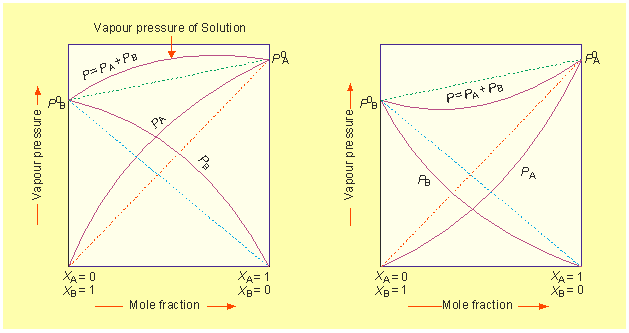These plots show how the vapour pressures of two component systems vary with their compositions

1. a solution that shows positive deviation from Raoult’s law and
2. a solution that shows negative deviation from Raoult’s

## Colligative Properties

(Latin word Colligative ? co = together, ligare = to bind.)

Colligative properties are the properties which are strictly dependent on the number of solute particles, but not on its nature.

about the examples of urea and sucrose where the vapour pressure of water is decreased by the same magnitude when equal molar mass of any compound is added to the fixed amount of water.

There are four types of colligative properties

(i) Relative lowering in vapour pressure (RLVP)

(ii) Elevation of boiling point (EBP)

(iii) Depression of freezing point (EFP)

(iv) Osmotic pressure (OP)

For describing all the four colligative properties, we assume a binary solution in which solvent and solute are denoted as 1 and 2 respectively.

(i) Relative lowering of vapour pressure (RLVP):-

We have already discussed that addition of non-volatile solute decreases the vapour pressure of solvent.

The lowering in vapour pressure is given by

p1o−p1 = p1ox2

Where, p1= Vapour pressure of pure solvent

p1 = Vapour pressure of solution when non-volatile solute is added.

x2 =mole fraction of solute.

From equation p1o−p1 = p1ox2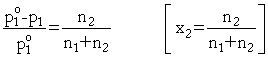Here, n1 and n2 are the number of moles of solvent and solute in the solution. For dilute solutions n2 << n1, neglecting n2 in the denominator we havew1 and w2 are masses and M1 and M2 are the molar masses of the solvent and solute respectively.

Here, we see that RLVP only depends on the mole fractions of solute, that is, the amount of solute. So this it is a colligative property.

Example 3: The vapour pressure of pure benzene at a certain temperature is 0.850 bar. A non-volatile, non-electrolyte solid weighing 0.5 g when added to 39.0 g of benzene (molar mass 78 g mol−1 ). Vapour pressure of the solution, then, is 0.845 bar. What is the molar mass of the solid substance?

Solution: The various quantities known to us are as follows:

p1o = 0.850 bar; = 0.845 bar; M1 = 78  mol−1, w2 = 0.5 g; w1= 39g

Substituting these values in equation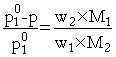, we getTherefore,  M= 170 g mol−1

(ii) Elevation in Boiling Point (EBP)

RLVP and EBP is interrelated. When the vapour pressure of a solution is decreased after addition of a non-volatile solute, its boiling point will automatically increase.

For example, if we take water, its normal boiling point is 100°C (i.e. 373 k). After addition of non-volatile solute to water its vapour pressure is decreased, it means at 373 k, its vapour is less than the atmospheric pressure. In order to increase the vapour pressure of this solution upto atmospheric pressure we have to supply more heat. This results in increase in boiling point of solution.

EBP can be expressed as ?Example 4: The boiling point of benzene is 353.23 K. When 1.80 g of a non-volatile solute was dissolved in 90 g of benzene, the boiling point is raised to 354.11 K. Calculate the molar mass of the solute, Kb for benzene is 2.53 k kg mol−1 .

Solution:

The elevation (ΔTb) in the boiling point = 354.11 K ? 353.23 K = 0.88 K. Substituting these values in expression ΔT= Kbm we get(iii) Depression in Freezing Point (DFP)

When the vapour pressure of a solution of its liquid phase becomes equal to the vapour pressure of its solid phase at a certain temperature, the liquid solution starts freezing and this temperature is called the freezing point of the solution.

After addition of a solute to a pure solvent, the freezing point of the solvent decreases, this is called depression in freezing point (ΔTf) .Where, ΔTf = depression in freezing point.

m = molality of the solution.

Kf = molal freezing point constant or Cryoscopic constant.

Kf is defined as the depression or lowering in the Freezing Point of 1 molal solution.

Example 5: 45 g of ethylene glycol (C2H6O2) is mixed with 600 g of water. Calculate (a) the freezing point depression and (b) the freezing point of the solution.

Solution: Depression in freezing point is related to the molality, therefore, the molality of the solution with respect to ethylene glycol =Freezing point of the aqueous solution = 273.15 K ? 2.2 K = 270.95 K

(iv) Osmotic Pressure (OP)

The tendency of a solvent to flow through a semipermeable membrane into a more concentrated solution is called Osmosis.

Semipermeable (semi = partially, Permiable = to pass through) membrane can only pass the solvent molecules but not the solute molecules into the solution. e.g. living cell, membrane, parchment paper etc.

When a pressure is applied to the solution side it is observed that the movement of solvent molecules into the solution side through semipermeable membrane is stopped. It means osmotic pressure is the extra pressure required to stop the movement of solvent molecules into solution side through SPM.

van?t Hoff showed osmotic pressure is related to the molar concentration of the solution as ?

or     λ    = MRT

λ    = (n2/v)RT

λ    = osmotic Pressure

M    = molarity of solution

R    = universal gas constant

T    = temperature in kelvin

n2    = number of moles of solute in solution

Out of the four colligative properties osmotic pressure is the best to calculate the molar mass of the solute (especially polymers, proteins) because ?

(i) it can be measured at room temperature.

(ii) ΔTf  and ΔTb are very small values and difficult to measure with ordinary temperature.

Example 6: 200 cm3 of an aqueous solution of a protein contains 1.26 g of the protein. The osmotic pressure of such a solution at 300 K is found to be 2.57 × 10−3 bar. Calculate the molar mass of the protein.

Solution: The various quantities known to us are as follows: λ = 2.57 × 10−3 bar,

V = 200cm3 = 0.200 litre

T = 300 K

R = 0.083 L bar mol1k−1KEY CONCEPT: Extraction of pure water from the water mixed with other solutes (for example sea water containing salts).

We know, in osmosis water (or solvent) moves into solution through semi permeable membrane.Here we see that when we apply the pressure more than osmotic pressure to the solution side only solvent squeezes out

But, it is possible when the pressure more than the osmotic pressure is applied to the solution side only solvent will squeeze out. This process is called reverse osmosis (see figure). Reverse osmosis is just opposite to the normal osmosis.

## Azeotropic Mixtures

A binary mixture

• that retains the same composition in the vapour state as in the liquid state when distilled or partially evaporated under a certain pressure.
• that cannot be separated by fractional distillation
• that has a constant boiling point and is called an azeotropic mixture or azeotrope.

Some azeotropes boil at lower boiling points than either of its constituents, called minimum boiling azeotropes. Maximum boiling azeotropes are those that boil at higher Boiling Point than either of its constituents Boiling Point.

Examples:

• Ethanol and water mixture (95%)
• Nitric acid and water mixture (68% m/m)

Minimum boiling azeotropes show positive deviation from Raoult?s law while maximum boiling azeotropes show negative deviation from Raoult’s law.

## Abnormal molar masses and colligative Properties

For the solute which undergo association or dissociation, observed value of colligative property is different from the calculated value of colligative property.

The ratio of observed colligative property to the calculated colligative property is known as van?t Hoff factor (i) ?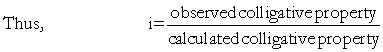Since the colligative property is directly related to the molecular mass of solute, for the solute which undergoes association or dissociation, abnormality in the molecular mass is observed.

Thus, also we can define i as,

Also,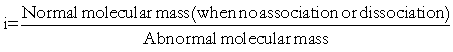Actually, abnormal molecular masses are experimentally determined when association or dissociation takes place.

Now, there may be three cases ?

(i) For a solute which doesn?t undergo any association or dissociation.

= 1

So, observed colligative property = calculated colligative property

e.g. Glucose, Urea, Sucrose etc. (when dissolved in water)

(ii) For a solute which undergoes dissociation, number of solute particles in solution increases and therefore

i > 1

so, observed colligative property > calculated Colligative Property

For example, if complete dissociation occurs then

i = 2 for KCl, NaNO3

i = 3 for CaCl2, Mg(NO3)2, Na2SO4

(iii) For solute which undergoes association, number of solute particles in solution decreases and therefore,

i < 1

and observed colligative property < calculated colligative properties.

In case of complete association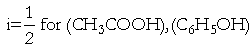Actually, CH3COOH or C6H5OH form dimmer and hence the number of solute particles becomes nearly half the initial number of solute.

2CH3COOH(CH3COOH)2

Example 7: The depression in freezing point observed when 0.6 mL of acetic acid (CH3COOH) , having density 1.06 g mL−1 , is dissolved in 1 litre of water is 0.0205°C . Calculate the van?t Hoff factor and the dissociation constant of acid.

Solution:Acetic acid is a weak electrolyte and will dissociate into two ions: acetate and hydrogen ions per molecule of acetic acid. If α is the degree of dissociation of acetic acid, then we would have n (1 − α) moles of undissociated acetic acid, nα moles of CH3COO and nα moles of H+ ions,

CH3COOHH+ CH3COO